# Determine the number of unpaired electrons, magnetic moment, and LFSE for following complexes: f. MnO4− g....

Determine the number of unpaired electrons, magnetic moment, and LFSE for following complexes:

f. MnO4−

g. Cu(H2O)6]2+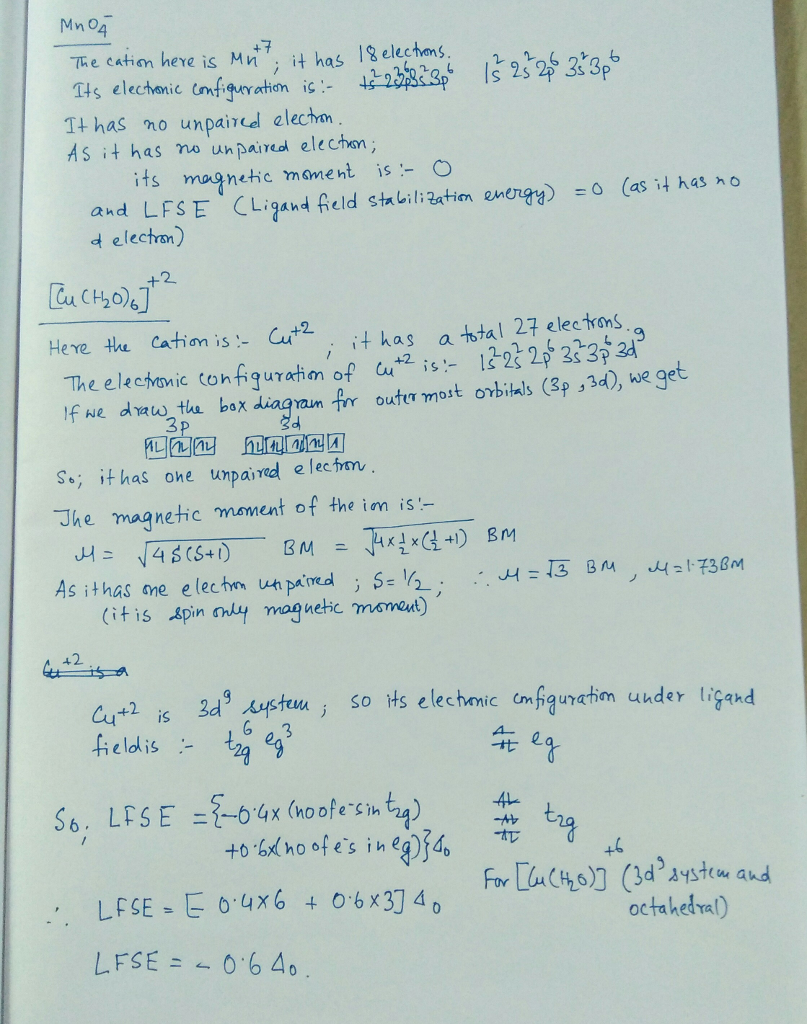##### Add Answer of: Determine the number of unpaired electrons, magnetic moment, and LFSE for following complexes: f. MnO4− g....
Similar Homework Help Questions
• ### (b) Determine the oxidation state of the metal, the d electron configuration, the number of unpaired electrons and the ligand field stabilisation energy for the following complexes: (i) [Co(NH3)5Br]2+...

(b) Determine the oxidation state of the metal, the d electron configuration, the number of unpaired electrons and the ligand field stabilisation energy for the following complexes: (i) [Co(NH3)5Br]2+ (ii) [MnO4]- (iii) [Fe(CN)6]3- (iv) [Cu(H2O)6]2+

• ### Calculate the spin only magnetic moment of each of the following complexes after determining the ...

Calculate the spin only magnetic moment of each of the following complexes after determining the of in unpaired electrons for each complex a) mercury tetrthiocyanatocobaltate (ii),b) tris(ethylenediamine)nickel(ii) thiosulphate and c) dichlorobis(triphenylphosphine) nickel (ii)

• ### 3 What spin only magnetic moment (uso) expected for each of the following complexes. (a) [MnBr.]...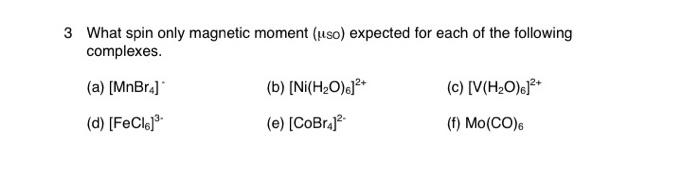3 What spin only magnetic moment (uso) expected for each of the following complexes. (a) [MnBr.] (C) [V(H2O)]2+ (b) [Ni(H20)]2+ (e) [CoBraj? (d) [FeC16] (f) Mo(CO)6

• ### All of the following complexes are either octahedral or tetrahedral. Determine if the complex is diamagnetic or par...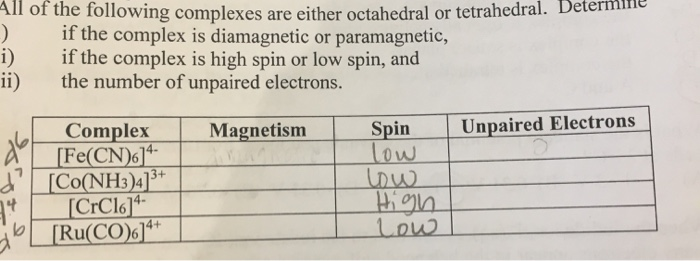All of the following complexes are either octahedral or tetrahedral. Determine if the complex is diamagnetic or paramagnetic, if the complex is high spin or low spin, and ii) the number of unpaired electrons. Unpaired Electrons Spin low Magnetism Complex [Fe(CN)6]* [Co(NH3)4]3 13+ 14- 4+ [Ru(CO)6] All of the following complexes are either octahedral or tetrahedral. Determine if the complex is diamagnetic or paramagnetic, if the complex is high spin or low spin, and ii) the number of unpaired electrons....

• ### Give the number of unpaired electrons in F-.

Give the number of unpaired electrons in F-.

• ### 3 What spin only magnetic moment (uso) expected for each of the following complexes. (a) [MnBra]...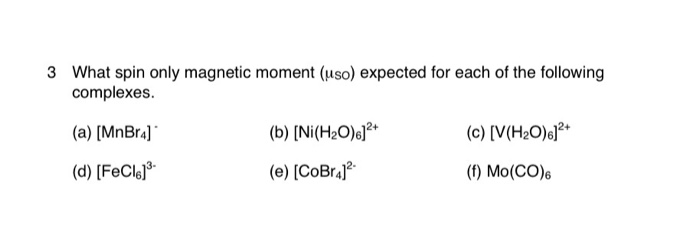3 What spin only magnetic moment (uso) expected for each of the following complexes. (a) [MnBra] (d) [FeC16] 19 NICOW" (c) [V(H2O)]2+ (b) [Ni(H2O)]2+ (e) [CoBraj? TAROT (1) Mo(CO)6

• ### For the following coordination compounds, use MO theory to predict the number of unpaired electrons in...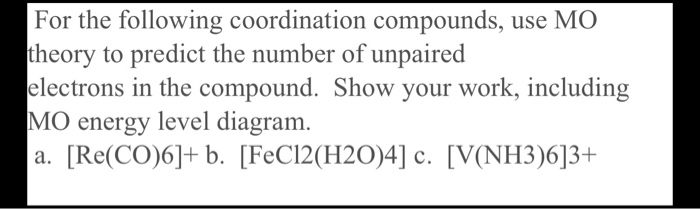For the following coordination compounds, use MO theory to predict the number of unpaired electrons in the compound. Show your work, including MO energy level diagram. a. [Re(CO)6]+ b. [FeC12(H2O)4] c. [V(NH3)6]3+

• ### Write abbreviated orbital notations for each of the following and determine the number of unpaired electrons in eac...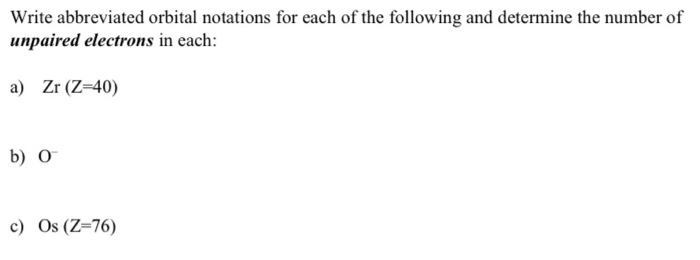Write abbreviated orbital notations for each of the following and determine the number of unpaired electrons in each: a) Zr (Z=40) b) o c) Os (Z=76)

• ### inorganic chemistry 2) A first-row transition metal complex of the formula (M(OH2)6]2+ has a magnetic moment...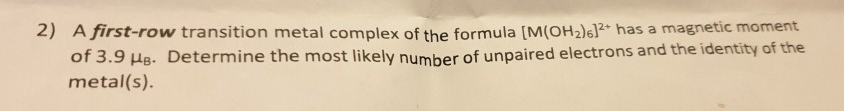inorganic chemistry 2) A first-row transition metal complex of the formula (M(OH2)6]2+ has a magnetic moment of 3.9 MR. Determine the most likely number of unpaired electrons and the identity of the metal(s).

• ### 6. Calculate the magnetic moment for the following complexes: a. (CrCl] [4% marks] b. [VC_04)2(NH3)21 [5%...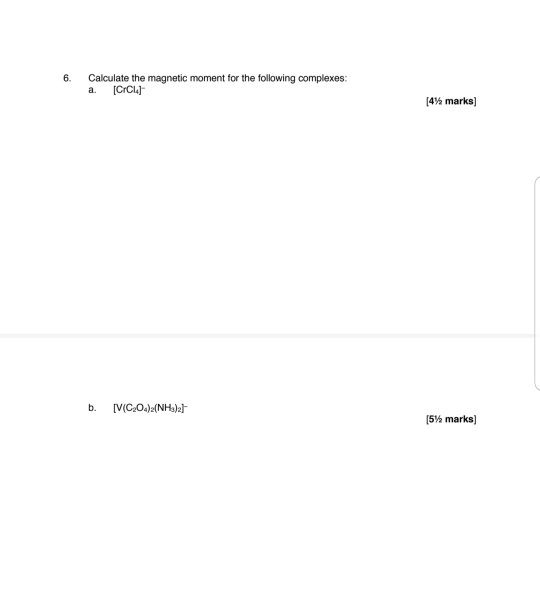6. Calculate the magnetic moment for the following complexes: a. (CrCl] [4% marks] b. [VC_04)2(NH3)21 [5% marks)

Need Online Homework Help?